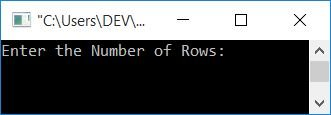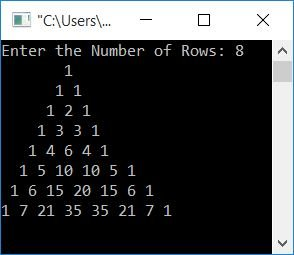# C Program to Print Pascal's Triangle

In this article, you will learn and get code for printing Pascal's triangle in C programming. But before going through the program, if you are not aware of Pascal's triangle, then I recommend you refer to the short description of Pascal's triangle. There, in a very short time, you will get everything that is required to create a program on it. But for now, the following picture tells everything about it.This figure defines Pascal's triangle. But I've written the simplest algorithm to create a Pascal's triangle in that article.

## Print Pascal's Triangle

Let's create a program to print Pascal's triangle without using any functions or formulas. This program only follows the algorithm to expand Pascal's triangle using loops and logic.

```#include<stdio.h>
#include<conio.h>
int main()
{
int row, col, i=1, j=0, arr, arrTemp;
arr = 1;
arr = 1;
for(row=0; row<5; row++)
{
for(col=4; col>row; col--)
printf(" ");
for(col=0; col<=row; col++)
{
if(row==0)
printf("1");
else
{
if(col==0 || col==row)
printf("1 ");
else
{
arrTemp[i] = arr[j]+arr[j+1];
printf("%d ", arrTemp[i]);
i++;
j++;
}
}
}
printf("\n");
arrTemp[i] = 1;
if(row>1)
{
j=0;
arr[j]=1;
for(j=1, i=1; j<=row; j++, i++)
arr[j] = arrTemp[i];
i=1;
j=0;
}
}
getch();
return 0;
}```

This program was built and runs in the Code::Blocks IDE. Here is its sample output.In the preceding program, we used two arrays so that the first array, arr[], holds the column's value for the previous row, and the second array, arrTemp[], holds the column's value for the next row. That is,

• Initially, 1 gets initialized to index 0 and 1 for the first array, say arr[].
• That is, arr = 1 and arr = 1.
• Using this array, the next row's column value is calculated in such a way that the values at the zeroth and first indexes are added and initialized to the first index of the second array, say arrTemp[].

The dry run of the above program goes like this:

1. Initially, i = 1, j = 0, arr  = 1, arr  = 1.
2. Using the for loop, 0 is now assigned to row (row = 0).
3. Determines whether or not it is less than 5. The condition evaluates to true, therefore program flow goes inside the loop. Inside it, using the first for loop, four spaces get printed.
4. The program flow now moves to the second for loop.
5. There, 0 is set to col (now col=0).
6. Checks whether col is less than or equal to the value of row or not.
7. The condition evaluates to true, therefore program flow goes inside this loop. Using an if statement, check whether the value of row is equal to 0 or not.
8. The condition evaluates to true, therefore program flow goes inside the if block and prints 1 on output. The else block gets skipped.
9. Now program flow goes to update part of the inner first for loop, incrementing the value of col.
10. Now col=1.
11. Process step no. 6 with the new value of col.
12. The condition returns false.
13. The next statement is
`printf("\n");`
This instructs the compiler to begin the next output items on the next or new line.
14. 1 is now initialized to arrTemp[i], because the previous value of i was 1. As a result, arrTemp = 1.
15. An if block is created to check whether the value of row is greater than 1 or not.
16. If the condition evaluates to false, the block is skipped.
17. And the program flow goes to the update part of the outer for loop. The value of "row" gets incremented.
18. Now row=1.
19. Process step no. 3 to 5.
20. Step 6 should be repeated with the new row value (which is 1).
21. Now col=0 once more.
23. The condition evaluates to false because row is not equal to 0.
24. As a result, program flow continues to another block.Inside the else block, it checks whether the value of col is equal to 0 or row's value or not.
25. Because its value is equal to 0, therefore 1, it gets printed.
26. it increases the value of col (now col = 1).
27. and determines whether it is less than or equal to row, condition evaluates to true, program flow again goes inside the loop.
28. Again the "else" block is executed.
29. And, once again, the if block condition evaluates to true because col (1) equals row (1).Therefore, 1 gets printed again.
30. Again, increments the value of col (now col = 2) and checks the condition. This time, the condition evaluates to false.
31. Process steps 13–17
32. Now row=2
33. Process step no. 3 to 5
34. Process step no. 6 with the new value of row (that is, 2).
35. Now col=0 once more.
36. Process steps no. 7, 23, 24, 25, 26, 27, and 28
37. Now col=2
38. This time, the condition of "if block" evaluates to false, therefore program flow goes to "else block."
39. There, arrTemp[i] = arr[j]+arr[j+1] or arrTemp = arr+arr[0+1] or arrTemp = 1+1 or arrTemp = 2
40. Now i = 2 and j = 1.
42. Now col=3
43. Process step no. 6 with the new value of col
44. Process step no. 12 to 15
45. The condition evaluates to true, therefore program flow goes inside the if block.
46. Now j = 0, arr[j] = 1, or arr = 1.
47. The for loop gets executed. after successfully executing it, we will have arr = 1, arr = 2, arr = 1.
48. Now i = 1 and j = 0.
49. Process step no. 17
50. Now row=3
51. The process is repeated from step 33 until the value of row equals 5. That is, the condition of the outer for loop evaluates to false.

## Using the Formula, print Pascal's Triangle

Now let's create another program that does the same job but uses a formula to find the column value one by one and gets printed directly without so much logic as given in the above program. But to improve programming skills, it is better to approach the previous one.

```#include<stdio.h>
#include<conio.h>
long int fact(int);
int main()
{
int i, c;
for(i=0; i<5; i++)
{
for(c=4; c>i; c--)
printf(" ");
for(c=0; c<=i; c++)
printf("%ld ", fact(i)/(fact(c)*fact(i-c)));
printf("\n");
}
getch();
return 0;
}
long int fact(int n)
{
int i, res=1;
for(i=1; i<=n; i++)
res = res*i;
return res;
}```

It will produce the same output as the previous one. The formula is given in the separate tutorial on Pascal's triangle. That is, the column value of every row of a Pascal's triangle can be calculated as:

`value = (row!)/((column!)*(row-columns)!)`

where row is the row number, and col is the column number.

Take note that both the row and the column begin at 0.

The ! represents factorial. To understand how to find the factorial of a number, refer to finding the factorial in C.

For example, the value in the 2nd column of the 4th row will be:

```value = (row!)/((column!)*(row-columns)!)
= (4!)/((2!)*(4-2)!)
= (24)/(2*(2!))
= 24/(2*2)
= 24/4
= 6```

So 6 is the number present at the fourth row and second column; that is actually at the fifth row and third column.

## Print Pascal's Triangle up to n rows

This program asks the user to define the size of Pascal's triangle, that is, how many rows he or she wants to print.

```#include<stdio.h>
#include<conio.h>
long int fact(int);
int main()
{
int i, c, rowLimit;
printf("Enter the Number of Rows: ");
scanf("%d", &rowLimit);
for(i=0; i<rowLimit; i++)
{
for(c=(rowLimit-1); c>i; c--)
printf(" ");
for(c=0; c<=i; c++)
printf("%ld ", fact(i)/(fact(c)*fact(i-c)));
printf("\n");
}
getch();
return 0;
}
long int fact(int n)
{
int i, res=1;
for(i=1; i<=n; i++)
res = res*i;
return res;
}```

Here is its sample run:Now supply the number of rows, that is, how many rows there are in Pascal's triangle to expand. Assume the user entered 8 as the number of rows and hit the ENTER key. Then here is the output produced by the above program:#### The same program in different languages

C Quiz

« Previous Program Next Program »